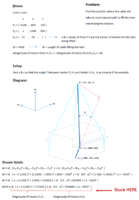Real World Lifting Problem

mmilez912

New member
I have a real world problem where I am trying to calculate the path a cable will take when lifting an item given certain dimensions. Basically the cable will have a starting (attach) point, and then travel past an edge with a given distance from center line in the y-dimension and z-dimension. I would like to calculate the x-dimension value that creates the straightest line possible (most natural path). The length of the cable is 4000mm. So in the following problem, the calculated x-dimension in P2 will affect the lifting point (z-dimension of P3)

I have tried using the distance formula and could solve for 1 variable or the other. I know to solve for 2 variables you must have 2 equations. I am just struggling to figure out what the second equation would be and hoping it doesn't involve calculus because I haven't taken it in like 15 years since college. lol. I'm assuming there's a derivative equation to figure out the minimum angle of T but I'm not sure what it would be.

Please review the following problem, diagram, and my shown work and any help would be greatly appreciated. Thank you so much for your time!!!